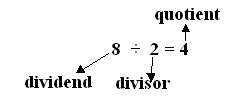Name: ___________________Date:___________________

 Email us to get an instant 20% discount on highly effective K-12 Math & English kwizNET Programs!

### Grade 3 - Mathematics10.4 Division Vocabulary

 Explanation: Addition, subtraction, multiplication and division are called operations. Subtraction is the inverse of addition. Multiplication is the inverse of division. Multiplication is repeated addition. Division is repeated subtraction. Zero divided by any number (except 0 equals 0) Number divided by any 0 is not defined Any number(except 0) divided by itself equals 1. Any number divided by 1 equals that number. The answer to the division problem is called the quotient. The number you are dividing is called the dividend. The number you are dividing by is called the divisor.Example: What times 5 equals 20? From 5 tables, we have 5 * 4 = 20 Answer: 4 Directions: Answer the following questions. Explain the division vocabulary in your own words. Also, write the times tables from one through ten.Name: ___________________Date:___________________

### Grade 3 - Mathematics10.4 Division Vocabulary

 Q 1: Any number (except 0) divided by itself equals 1.FalseTrue Q 2: You cannot divide by 0 (ex: 5/0 is impossible).TrueFalse Q 3: Any number divided by the same number is equal to 1.TrueFalse Q 4: What times 4 equals 28?867 Q 5: What times 5 equals 10?1432 Q 6: Any number divided by 1 equals that number.TrueFalse Q 7: 0 divided by any number (except 0) equalsthe same number10 Q 8: For 6 x 7 = 42, the corresponding division fact is42/7 = 66/7 = 426/7 = 42 Question 9: This question is available to subscribers only! Question 10: This question is available to subscribers only!